# Chemical equilibrium

17 de Jan de 2011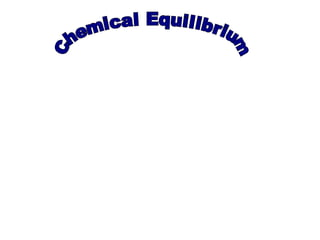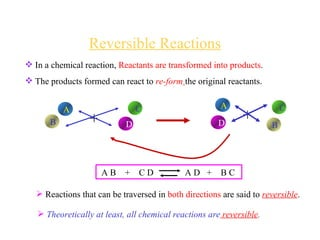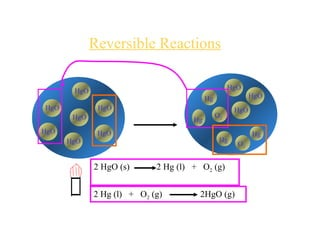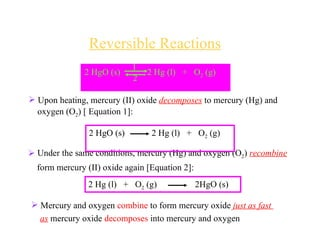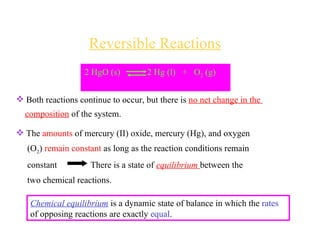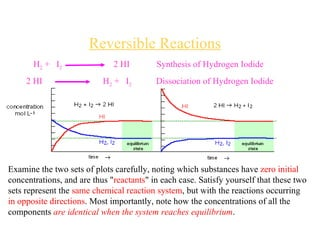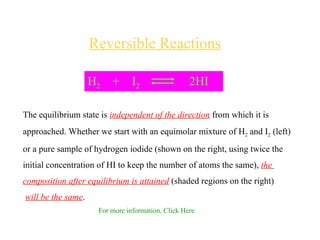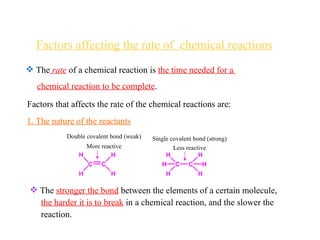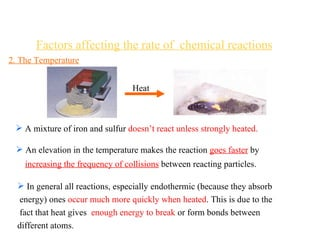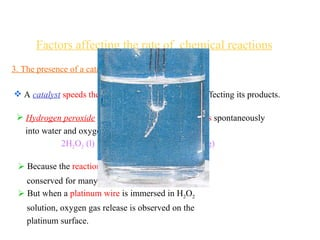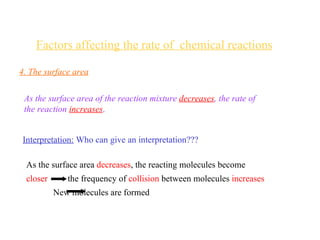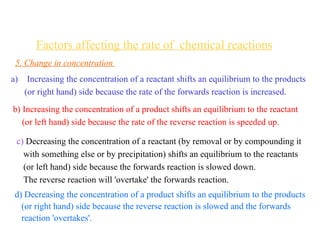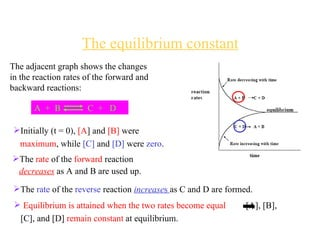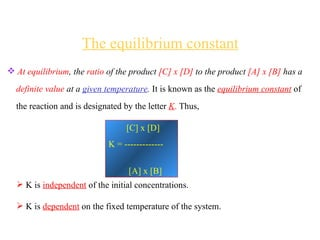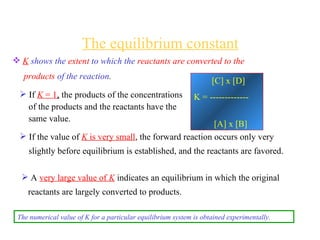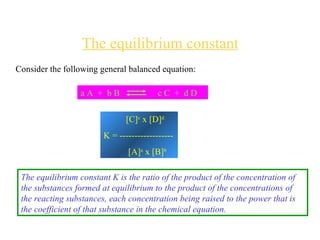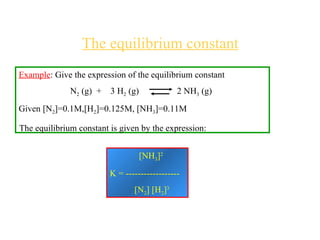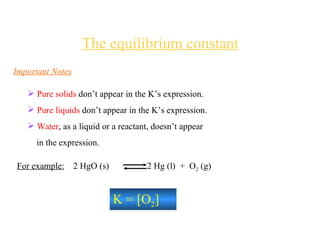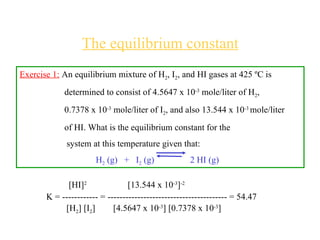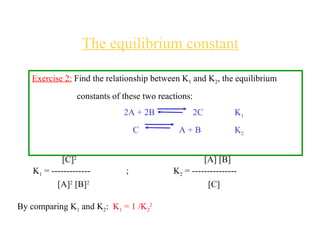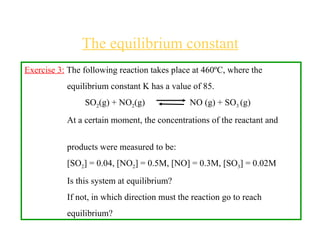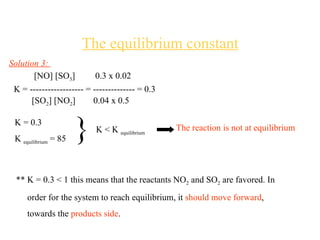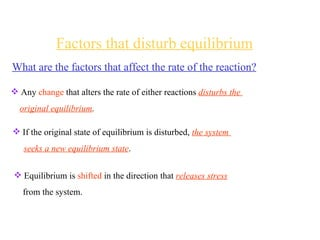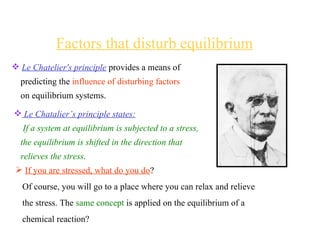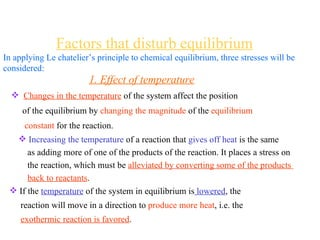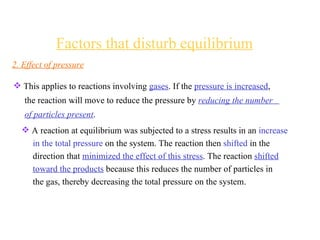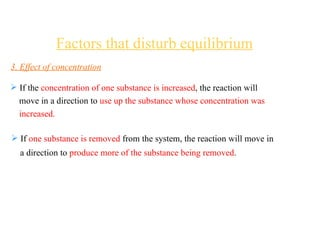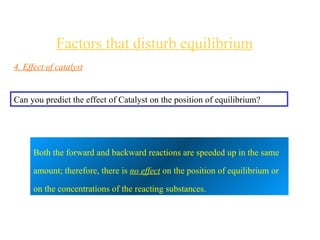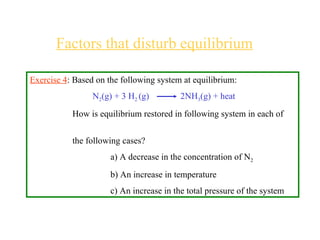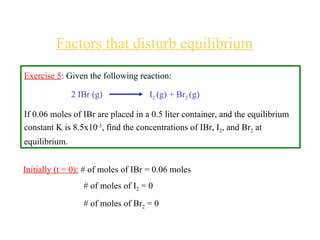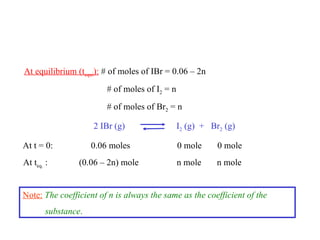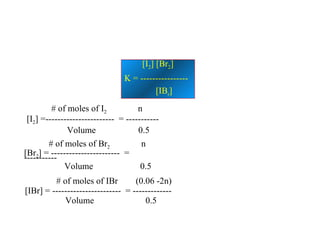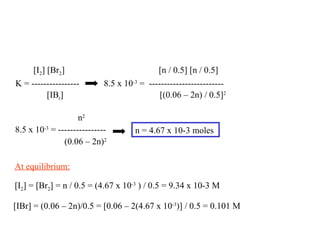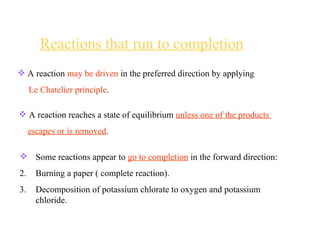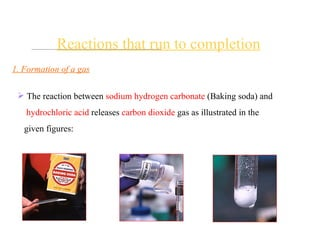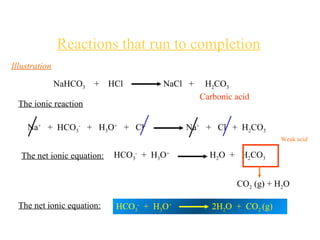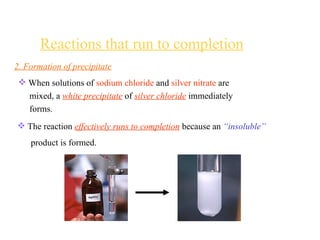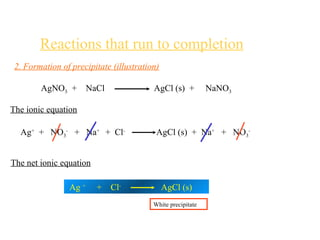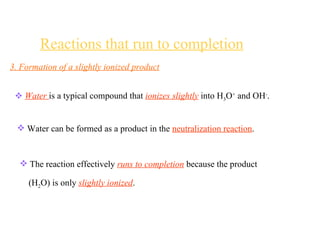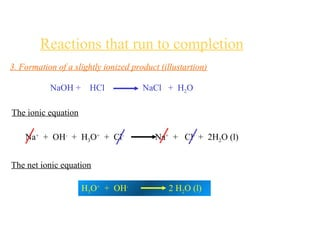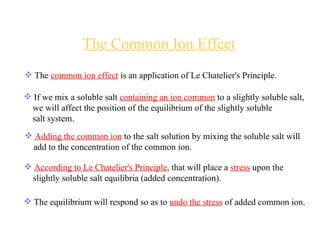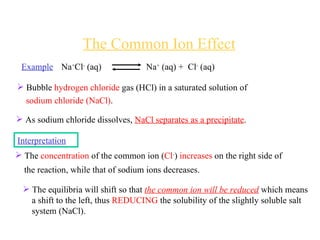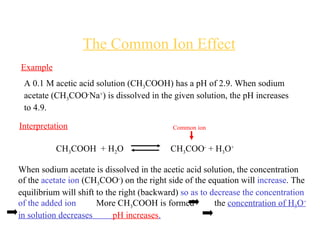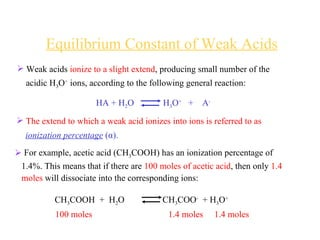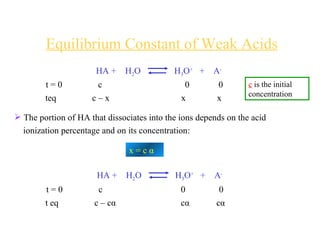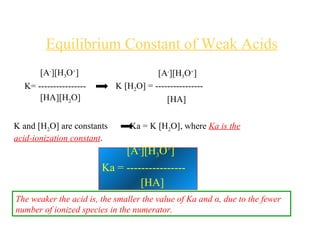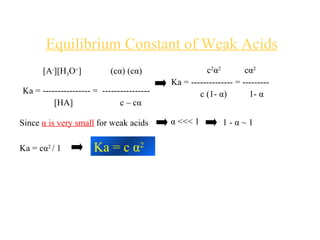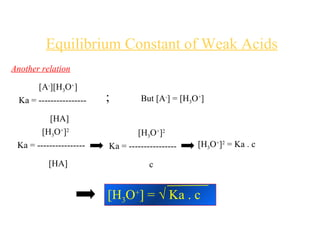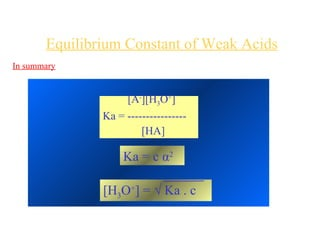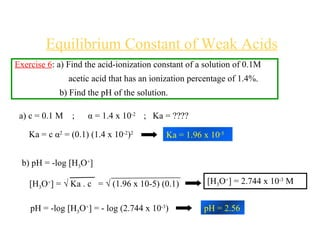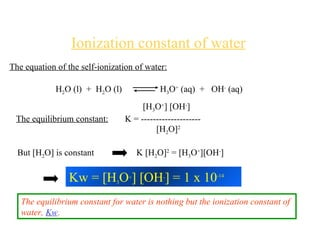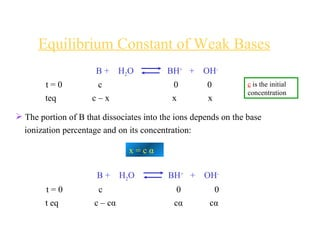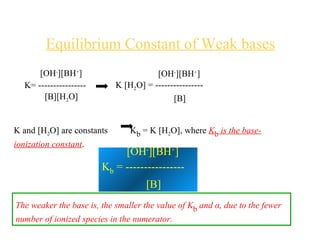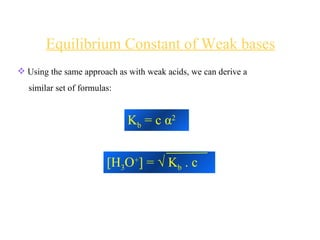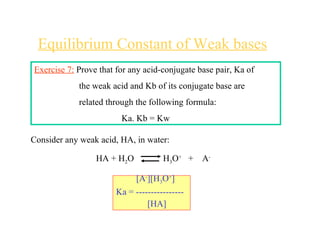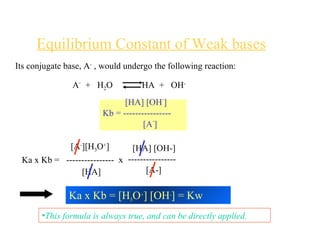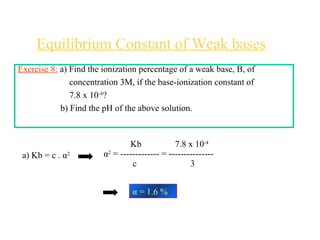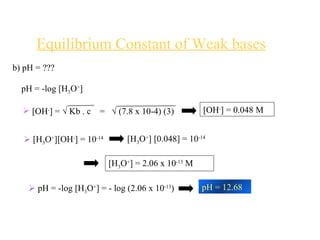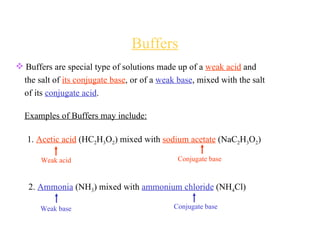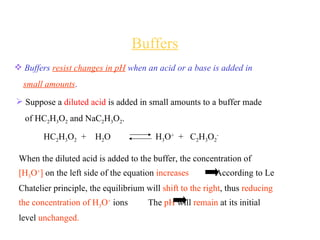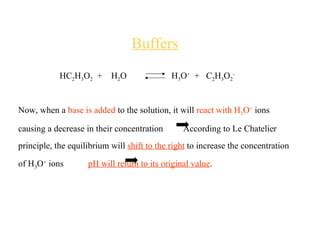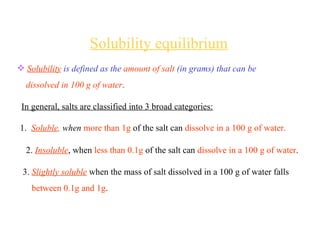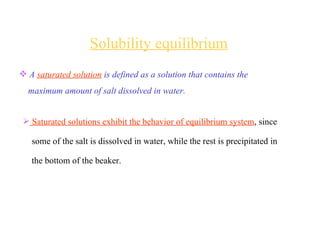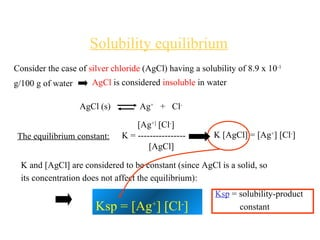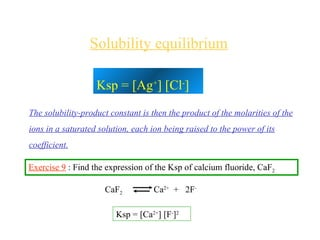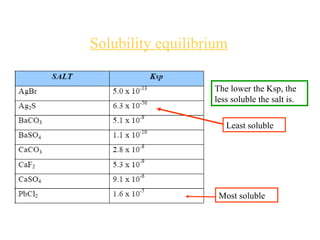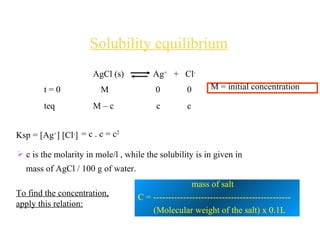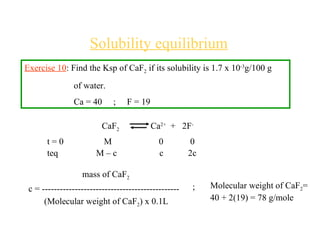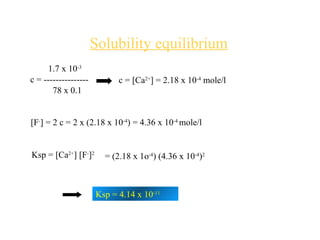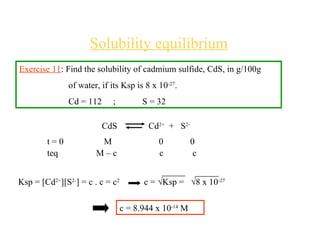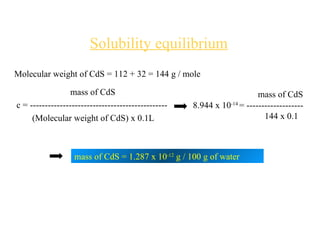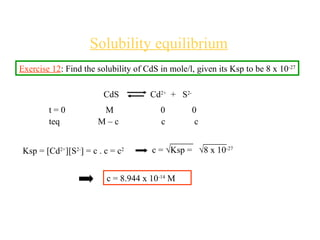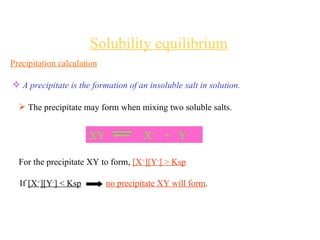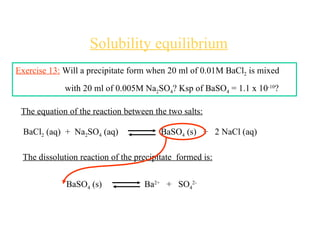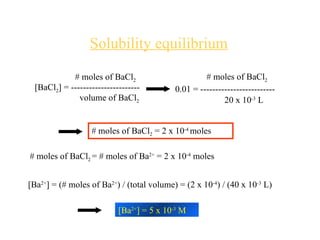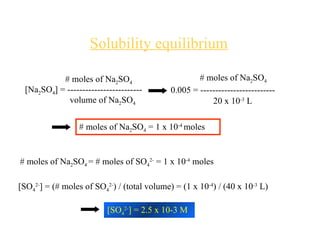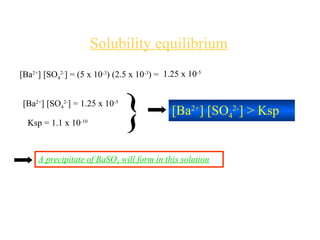1 de 77

### Chemical equilibrium

• 1. Chemical Equilibrium
• 2.
• 3. Reversible Reactions HgO HgO HgO HgO HgO HgO HgO Hg O 2 Hg Hg HgO Hg HgO O 2 HgO 2 HgO (s) 2 Hg (l) + O 2 (g) 2 Hg (l) + O 2 (g) 2HgO (g)
• 4.
• 5.
• 6. Reversible Reactions Examine the two sets of plots carefully, noting which substances have zero initial concentrations, and are thus &quot; reactants &quot; in each case. Satisfy yourself that these two sets represent the same chemical reaction system , but with the reactions occurring in opposite directions . Most importantly, note how the concentrations of all the components are identical when the system reaches equilibrium . 2 HI H 2 + I 2 Dissociation of Hydrogen Iodide H 2 + I 2 2 HI Synthesis of Hydrogen Iodide
• 7. Reversible Reactions The equilibrium state is independent of the direction from which it is approached. Whether we start with an equimolar mixture of H 2 and I 2 (left) or a pure sample of hydrogen iodide (shown on the right, using twice the initial concentration of HI to keep the number of atoms the same), the composition after equilibrium is attained (shaded regions on the right) will be the same . H 2 + I 2 2HI For more information, Click Here
• 8.
• 9.
• 10.
• 11. Factors affecting the rate of chemical reactions 4. The surface area As the surface area of the reaction mixture decreases , the rate of the reaction increases . Interpretation: Who can give an interpretation??? As the surface area decreases , the reacting molecules become closer the frequency of collision between molecules increases New molecules are formed
• 12.
• 13.
• 14.
• 15.
• 16. The equilibrium constant Consider the following general balanced equation: a A + b B c C + d D [C] c x [D] d K = ------------------ [A] a x [B] b The equilibrium constant K is the ratio of the product of the concentration of the substances formed at equilibrium to the product of the concentrations of the reacting substances, each concentration being raised to the power that is the coefficient of that substance in the chemical equation.
• 17. The equilibrium constant Example : Give the expression of the equilibrium constant N 2 (g) + 3 H 2 (g) 2 NH 3 (g) Given [N 2 ]=0.1M,[H 2 ]=0.125M, [NH 3 ]=0.11M The equilibrium constant is given by the expression: [NH 3 ] 2 K = ------------------ [N 2 ] [H 2 ] 3
• 18.
• 19. The equilibrium constant Exercise 1: An equilibrium mixture of H 2 , I 2 , and HI gases at 425 ºC is determined to consist of 4.5647 x 10 -3 mole/liter of H 2 , 0.7378 x 10 -3 mole/liter of I 2 , and also 13.544 x 10 -3 mole/liter of HI. What is the equilibrium constant for the system at this temperature given that: H 2 (g) + I 2 (g) 2 HI (g) [HI] 2 [13.544 x 10 -3 ] -2 K = ------------ = ---------------------------------------- = 54.47 [H 2 ] [I 2 ] [4.5647 x 10 -3 ] [0.7378 x 10 -3 ]
• 20. The equilibrium constant Exercise 2: Find the relationship between K 1 and K 2 , the equilibrium constants of these two reactions: 2A + 2B 2C K 1 C A + B K 2 [C] 2 [A] [B] K 1 = ------------- ; K 2 = --------------- [A] 2 [B] 2 [C] By comparing K 1 and K 2 : K 1 = 1 /K 2 2
• 21. The equilibrium constant Exercise 3: The following reaction takes place at 460ºC, where the equilibrium constant K has a value of 85. SO 2 (g) + NO 2 (g) NO (g) + SO 3 (g) At a certain moment, the concentrations of the reactant and products were measured to be: [SO 2 ] = 0.04, [NO 2 ] = 0.5M, [NO] = 0.3M, [SO 3 ] = 0.02M Is this system at equilibrium? If not, in which direction must the reaction go to reach equilibrium?
• 22. The equilibrium constant Solution 3: [NO] [SO 3 ] 0.3 x 0.02 K = ------------------ = -------------- = 0.3 [SO 2 ] [NO 2 ] 0.04 x 0.5 K = 0.3 K equilibrium = 85 } K < K equilibrium The reaction is not at equilibrium ** K = 0.3 < 1 this means that the reactants NO 2 and SO 2 are favored. In order for the system to reach equilibrium, it should move forward , towards the products side .
• 23.
• 24.
• 25.
• 26.
• 27.
• 28. Factors that disturb equilibrium 4. Effect of catalyst Can you predict the effect of Catalyst on the position of equilibrium? Both the forward and backward reactions are speeded up in the same amount; therefore, there is no effect on the position of equilibrium or on the concentrations of the reacting substances.
• 29. Factors that disturb equilibrium Exercise 4 : Based on the following system at equilibrium: N 2 (g) + 3 H 2 (g) 2NH 3 (g) + heat How is equilibrium restored in following system in each of the following cases? a) A decrease in the concentration of N 2 b) An increase in temperature c) An increase in the total pressure of the system
• 30. Factors that disturb equilibrium Exercise 5 : Given the following reaction: 2 IBr (g) I 2 (g) + Br 2 (g) If 0.06 moles of IBr are placed in a 0.5 liter container, and the equilibrium constant K is 8.5x10 -3 , find the concentrations of IBr, I 2 , and Br 2 at equilibrium. Initially (t = 0): # of moles of IBr = 0.06 moles # of moles of I 2 = 0 # of moles of Br 2 = 0
• 31. At equilibrium (t equi ): # of moles of IBr = 0.06 – 2n # of moles of I 2 = n # of moles of Br 2 = n 2 IBr (g) I 2 (g) + Br 2 (g) At t = 0: 0.06 moles 0 mole 0 mole At t eq. : (0.06 – 2n) mole n mole n mole Note: The coefficient of n is always the same as the coefficient of the substance .
• 32. [I 2 ] [Br 2 ] K = ---------------- [IB r ] # of moles of I 2 n [I 2 ] =----------------------- = ----------- Volume 0.5 # of moles of Br 2 n [Br 2 ] = ----------------------- = ----------- Volume 0.5 # of moles of IBr (0.06 -2n) [IBr] = ----------------------- = ------------- Volume 0.5
• 33. [I 2 ] [Br 2 ] [n / 0.5] [n / 0.5] K = ---------------- 8.5 x 10 -3 = ------------------------- [IB r ] [(0.06 – 2n) / 0.5] 2 n 2 8.5 x 10 -3 = ---------------- (0.06 – 2n) 2 n = 4.67 x 10-3 moles [I 2 ] = [Br 2 ] = n / 0.5 = (4.67 x 10 -3 ) / 0.5 = 9.34 x 10-3 M [IBr] = (0.06 – 2n)/0.5 = [0.06 – 2(4.67 x 10 -3 )] / 0.5 = 0.101 M At equilibrium:
• 34.
• 35.
• 36. Reactions that run to completion Illustration NaHCO 3 + HCl NaCl + H 2 CO 3 The ionic reaction Na + + HCO 3 - + H 3 O + + Cl - Na + + Cl - + H 2 CO 3 The net ionic equation: HCO 3 - + H 3 O + H 2 O + H 2 CO 3 CO 2 (g) + H 2 O The net ionic equation: HCO 3 - + H 3 O + 2H 2 O + CO 2 (g) Weak acid Carbonic acid
• 37.
• 38. Reactions that run to completion 2. Formation of precipitate (illustration) AgNO 3 + NaCl AgCl (s) + NaNO 3 The ionic equation Ag + + NO 3 - + Na + + Cl - AgCl (s) + Na + + NO 3 - The net ionic equation Ag + + Cl - AgCl (s) White precipitate
• 39.
• 40. Reactions that run to completion 3. Formation of a slightly ionized product (illustartion) NaOH + HCl NaCl + H 2 O The ionic equation Na + + OH - + H 3 O + + Cl - Na + + Cl - + 2H 2 O (l) The net ionic equation H 3 O + + OH - 2 H 2 O (l)
• 41.
• 42.
• 43. The Common Ion Effect Example A 0.1 M acetic acid solution (CH 3 COOH) has a pH of 2.9. When sodium acetate (CH 3 COO - Na + ) is dissolved in the given solution, the pH increases to 4.9. Interpretation CH 3 COOH + H 2 O CH 3 COO - + H 3 O + When sodium acetate is dissolved in the acetic acid solution, the concentration of the acetate ion (CH 3 COO - ) on the right side of the equation will increase . The equilibrium will shift to the right (backward) so as to decrease the concentration of the added ion More CH 3 COOH is formed the concentration of H 3 O + in solution decreases pH increases . Common ion
• 44.
• 45.
• 46. [A - ][H 3 O + ] K= ---------------- [HA][H 2 O] [A - ][H 3 O + ] K [H 2 O] = ---------------- [HA] K and [H 2 O] are constants Ka = K [H 2 O], where Ka is the acid-ionization constant . [A - ][H 3 O + ] Ka = ---------------- [HA] The weaker the acid is, the smaller the value of Ka and α, due to the fewer number of ionized species in the numerator. Equilibrium Constant of Weak Acids
• 47. Equilibrium Constant of Weak Acids [A - ][H 3 O + ] (c α ) (c α ) Ka = ---------------- = ---------------- [HA] c – c α c 2 α 2 c α 2 Ka = -------------- = --------- c (1- α ) 1- α Since α is very small for weak acids α <<< 1 1 - α ~ 1 Ka = c α 2 / 1 Ka = c α 2
• 48. Equilibrium Constant of Weak Acids Another relation [A - ][H 3 O + ] Ka = ---------------- [HA] But [A - ] = [H 3 O + ] ; [H 3 O + ] 2 Ka = ---------------- [HA] [H 3 O + ] 2 Ka = ---------------- c [H 3 O + ] 2 = Ka . c [H 3 O + ] = √ Ka . c
• 49. Equilibrium Constant of Weak Acids In summary [H 3 O + ] = √ Ka . c Ka = c α 2 [A - ][H 3 O + ] Ka = ---------------- [HA]
• 50. Equilibrium Constant of Weak Acids Exercise 6 : a) Find the acid-ionization constant of a solution of 0.1M acetic acid that has an ionization percentage of 1.4%. b) Find the pH of the solution. a) c = 0.1 M ; α = 1.4 x 10 -2 ; Ka = ???? Ka = c α 2 = (0.1) (1.4 x 10 -2 ) 2 Ka = 1.96 x 10 -5 b) pH = -log [H 3 O + ] [H 3 O + ] = √ Ka . c = √ (1.96 x 10-5) (0.1) [H 3 O + ] = 2.744 x 10 -3 M pH = -log [H 3 O + ] = - log (2.744 x 10 -3 ) pH = 2.56
• 51. Ionization constant of water The equation of the self-ionization of water: H 2 O (l) + H 2 O (l) H 3 O + (aq) + OH - (aq) The equilibrium constant: [H 3 O + ] [OH - ] K = -------------------- [H 2 O] 2 But [H 2 O] is constant K [H 2 O] 2 = [H 3 O + ][OH - ] Kw = [H 3 O + ] [OH - ] = 1 x 10 -14 The equilibrium constant for water is nothing but the ionization constant of water, Kw .
• 52.
• 53. [OH - ][BH + ] K= ---------------- [B][H 2 O] [OH - ][BH + ] K [H 2 O] = ---------------- [B] K and [H 2 O] are constants K b = K [H 2 O], where K b is the base-ionization constant . [OH - ][BH + ] K b = ---------------- [B] The weaker the base is, the smaller the value of K b and α, due to the fewer number of ionized species in the numerator. Equilibrium Constant of Weak bases
• 54.
• 55. Equilibrium Constant of Weak bases Exercise 7: Prove that for any acid-conjugate base pair, Ka of the weak acid and Kb of its conjugate base are related through the following formula: Ka. Kb = Kw Consider any weak acid, HA, in water: HA + H 2 O H 3 O + + A - [A - ][H 3 O + ] Ka = ---------------- [HA]
• 56.
• 57. Equilibrium Constant of Weak bases Exercise 8: a) Find the ionization percentage of a weak base, B, of concentration 3M, if the base-ionization constant of 7.8 x 10 -4 ? b) Find the pH of the above solution. a) Kb = c . α 2 Kb 7.8 x 10 -4 α 2 = ------------- = --------------- c 3 α = 1.6 %
• 58.
• 59.
• 60.
• 61. HC 2 H 3 O 2 + H 2 O H 3 O + + C 2 H 3 O 2 - Now, when a base is added to the solution, it will react with H 3 O + ions causing a decrease in their concentration According to Le Chatelier principle, the equilibrium will shift to the right to increase the concentration of H 3 O + ions pH will return to its original value . Buffers
• 62.
• 63.
• 64. Solubility equilibrium Consider the case of silver chloride (AgCl) having a solubility of 8.9 x 10 -1 g/100 g of water AgCl is considered insoluble in water AgCl (s) Ag + + Cl - The equilibrium constant: [Ag +] [Cl - ] K = ---------------- [AgCl] K [AgCl] = [Ag + ] [Cl - ] K and [AgCl] are considered to be constant (since AgCl is a solid, so its concentration does not affect the equilibrium): Ksp = [Ag + ] [Cl - ] Ksp = solubility-product constant
• 65. Solubility equilibrium The solubility-product constant is then the product of the molarities of the ions in a saturated solution, each ion being raised to the power of its coefficient. Ksp = [Ag + ] [Cl - ] Exercise 9 : Find the expression of the Ksp of calcium fluoride, CaF 2 CaF 2 Ca 2+ + 2F - Ksp = [Ca 2+ ] [F - ] 2
• 66. Solubility equilibrium The lower the Ksp, the less soluble the salt is. Least soluble Most soluble
• 67.
• 68. Solubility equilibrium Exercise 10 : Find the Ksp of CaF 2 if its solubility is 1.7 x 10 -3 g/100 g of water. Ca = 40 ; F = 19 CaF 2 Ca 2+ + 2F - t = 0 M 0 0 teq M – c c 2c mass of CaF 2 c = ---------------------------------------------- (Molecular weight of CaF 2 ) x 0.1L Molecular weight of CaF 2 = 40 + 2(19) = 78 g/mole ;
• 69. Solubility equilibrium 1.7 x 10 -3 c = --------------- 78 x 0.1 c = [Ca 2+ ] = 2.18 x 10 -4 mole/l [F - ] = 2 c = 2 x (2.18 x 10 -4 ) = 4.36 x 10 -4 mole/l Ksp = [Ca 2+ ] [F - ] 2 = (2.18 x 1o -4 ) (4.36 x 10 -4 ) 2 Ksp = 4.14 x 10 -11
• 70. Solubility equilibrium Exercise 11 : Find the solubility of cadmium sulfide, CdS, in g/100g of water, if its Ksp is 8 x 10 -27 . Cd = 112 ; S = 32 CdS Cd 2+ + S 2- t = 0 M 0 0 teq M – c c c Ksp = [Cd 2+ ][S 2- ] = c . c = c 2 c = √Ksp = √8 x 10 -27 c = 8.944 x 10 -14 M
• 71. mass of CdS c = ---------------------------------------------- (Molecular weight of CdS) x 0.1L Molecular weight of CdS = 112 + 32 = 144 g / mole mass of CdS 8.944 x 10 -14 = ------------------- 144 x 0.1 mass of CdS = 1.287 x 10 -12 g / 100 g of water Solubility equilibrium
• 72. Solubility equilibrium Exercise 12 : Find the solubility of CdS in mole/l, given its Ksp to be 8 x 10 -27 Ksp = [Cd 2+ ][S 2- ] = c . c = c 2 CdS Cd 2+ + S 2- t = 0 M 0 0 teq M – c c c c = √Ksp = √8 x 10 -27 c = 8.944 x 10 -14 M
• 73.
• 74. Solubility equilibrium Exercise 13: Will a precipitate form when 20 ml of 0.01M BaCl 2 is mixed with 20 ml of 0.005M Na 2 SO 4 ? Ksp of BaSO 4 = 1.1 x 10 -10 ? BaCl 2 (aq) + Na 2 SO 4 (aq) BaSO 4 (s) + 2 NaCl (aq) The equation of the reaction between the two salts: The dissolution reaction of the precipitate formed is: BaSO 4 (s) Ba 2+ + SO 4 2-
• 75. Solubility equilibrium [Ba 2+ ] = 5 x 10 -3 M # moles of BaCl 2 = 2 x 10 -4 moles # moles of BaCl 2 = # moles of Ba 2+ = 2 x 10 -4 moles [Ba 2+ ] = (# moles of Ba 2+ ) / (total volume) = (2 x 10 -4 ) / (40 x 10 -3 L) # moles of BaCl 2 [BaCl 2 ] = ----------------------- volume of BaCl 2 # moles of BaCl 2 0.01 = ------------------------- 20 x 10 -3 L
• 76. Solubility equilibrium # moles of Na 2 SO 4 = # moles of SO 4 2- = 1 x 10 -4 moles [SO 4 2- ] = (# moles of SO 4 2- ) / (total volume) = (1 x 10 -4 ) / (40 x 10 -3 L) [SO 4 2- ] = 2.5 x 10-3 M # moles of Na 2 SO 4 [Na 2 SO 4 ] = ------------------------- volume of Na 2 SO 4 # moles of Na 2 SO 4 0.005 = ------------------------- 20 x 10 -3 L # moles of Na 2 SO 4 = 1 x 10 -4 moles
• 77. Solubility equilibrium [Ba 2+ ] [SO 4 2- ] = (5 x 10 -3 ) (2.5 x 10 -3 ) = 1.25 x 10 -5 [Ba 2+ ] [SO 4 2- ] = 1.25 x 10 -5 Ksp = 1.1 x 10 -10 } [Ba 2+ ] [SO 4 2- ] > Ksp A precipitate of BaSO 4 will form in this solution